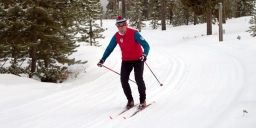# Half-circles 81731

A skier skis down a black slope. He makes a total of 31 half-circles while going down the hill. The radius of one semicircle is 4m. What distance did he travel?

x =  390 m

### Step-by-step explanation:Did you find an error or inaccuracy? Feel free to write us. Thank you!

Tips for related online calculators
The line slope calculator is helpful for basic calculations in analytic geometry. The coordinates of two points in the plane calculate slope, normal and parametric line equation(s), slope, directional angle, direction vector, the length of the segment, intersections of the coordinate axes, etc.

#### Grade of the word problem:

We encourage you to watch this tutorial video on this math problem: